Courses

# NCERT Exemplar - D and F - Block Elements NEET Notes | EduRev

## JEE Revision Notes

Created by: Mohit Rajpoot

## NEET : NCERT Exemplar - D and F - Block Elements NEET Notes | EduRev

The document NCERT Exemplar - D and F - Block Elements NEET Notes | EduRev is a part of the NEET Course JEE Revision Notes.
All you need of NEET at this link: NEET

MULTIPLE CHOICE QUESTIONS (TYPE - I)

Q.1. Electronic configuration of a transition element X in +3 oxidation state is [Ar]3d5. What is its atomic number?
(i) 25
(ii) 26
(iii) 27
(iv) 24
Ans. (ii)
Solution.
Electronic configuration of X3+ is [Ar] 3d5
It represents the total number of electrons and oxidation state.
Therefore, atomic number of X = 18+ 5+ 3 = 26
Hence, option (ii) is correct.

Q.2. The electronic configuration of Cu(II) is 3d9 whereas that of Cu(I) is 3d10.  Which of the following is correct?
(i) Cu(II) is more stable
(ii) Cu(II) is less stable
(iii) Cu(I) and Cu(II) are equally stable
(iv) Stability of Cu(I) and Cu(II) depends on nature of copper salts
Ans. (i)
Solution.

Cu (II) is more stable than Cu (I). As it is known that, Cu (I) has 3d10 stable configuration while Cu (II) has 3d9 configuration. But Cu (II) is more stable due to greater effective nuclear, i.e., it hold 17 electrons instead of 18 in Cu (I).

Q.3. Metallic radii of some transition elements are given below. Which of these elements will have highest density?

 Element Fe Co Ni Cu Metallic radii/pm 126 125 125 128

(i) Fe
(ii) Ni
(iii) Co
(iv) Cu

Ans. (iv)
Solution.

On moving left to right along a period, metallic radius decreases while mass increases.
Decrease in metallic radius coupled with increase in atomic mass results in increase in density of metal.
Hence, among the given four choices Cu belongs to right side of Periodic Table in transition metal, and it has highest density (89 g/cm3).

Q.4. Generally transition elements form coloured salts due to the presence of unpaired electrons. Which of the following compounds will be coloured in solid state?
(i) Ag2SO4
(ii) CuF2
(iii) ZnF2
(iv) Cu2Cl2
Ans. (ii)
Solution.

Transition elements form coloured salt due to the presence of unpaired electrons. In CuF2, Cu (II) contains one unpaired electron hence, CuF2 is coloured in solid state.

Q.5. On addition of small amount of KMnO4 to concentrated H2SO4, a green oily compound is obtained which is highly explosive in nature. Identify the compound from the following.
(i) Mn2O7
(ii) MnO2
(iii) MnSO4
(iv) Mn2O3
Ans. (i)
Solution.

On addition of KMnO4 to concentrated H2SO4, a green oily compound Mn2O7 is obtained which is highly explosive in nature.
2KMnO4 + 2H2SO4 (Conc.) → Mn2O+ 2KHSO4 + H2O

Q.6. The magnetic nature of elements depends on the presence of unpaired electrons. Identify the configuration of transition element, which shows highest magnetic moment.
(i) 3d7
(ii) 3d5
(iii) 3d8
(iv) 3d2
Ans. (ii)
Solution.

Greater the number of unpaired electrons, higher will its value of magnetic moment. Since, 3d5 has 5 unpaired electrons hence, it has the highest magnetic moment.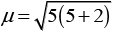= 5.95BM

Q.7. Which of the following oxidation state is common for all lanthanoids?
(i) +2
(ii) +3
(iii) +4
(iv) +5
Ans. (ii)
Solution.

Lanthanoids show common oxidation state of +3. Some of the lanthanoids also show + 2 and +4 stable oxidation state along with +3 oxidation state. These are shown by those elements which by losing 2 or 4 electrons acquire a stable configuration of f0, f7 or f14,e.g.,
Eu2+ is [Xe] 4f7, Yb2+ is [Xe]4f14, Ce4+ is [Xe]4f° and Tb4f is [Xe]4f7

Q.8. Which of the following reactions are disproportionation reactions?
(a) Cu+ → Cu2+ + Cu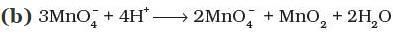(c) 2KMnO4 → K2MnO4  + MnO2 + O2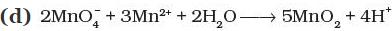(i) a
(ii) a, b, c
(iii) b, c, d
(iv) a, d
Ans. (i)
Solution.

The reaction in which same element is simultaneously oxidized and reduced is termed as disproportionation reaction.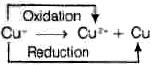Q.9. When KMnO4 solution is added to oxalic acid solution, the decolourisation is slow in the beginning but becomes instantaneous after some time because
(i) CO2 is formed as the product.
(ii) Reaction is exothermic.
(iii)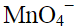catalyses the reaction.
(iv) Mn2+ acts as autocatalyst.
Ans. (iv)
Solution.

When KMnO4 solution is added to oxalic acid solution, the decolourisation is slow in the beginning but becomes instantaneous after some time because Mn2+ acts as autocatalyst.
Reduction half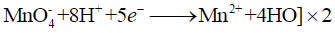Oxidation half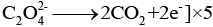Overall equation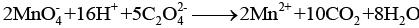End point of this reaction Colourless to light pink

Q.10. There are 14 elements in actinoid series. Which of the following elements does not belong to this series?
(i) U
(ii) Np
(iii) Tm
(iv) Fm
Ans. (iii)
Solution.

Tm (Z = 69) does not belong to actinoid series. The actinoid series is with atomic numbers 90 to 103. Thulium (Tm) has atomic number 69 belongs to lanthanoids (4 f series).

Q.11. KMnO4 acts as an oxidising agent in acidic medium. The number of moles of KMnO4 that will be needed to react with one mole of sulphide ions in acidic solution is
(i) 2/5
(ii) 3/5
(iii) 4/5
(iv) 1/5
Ans. (i)
Solution.

The reaction of KMnOin which it acts as an oxidising agent in acidic medium is
2KMnO4 + 3H2SO4 → K2SO4 + 2MnSO4 + 3H2O + 5[O]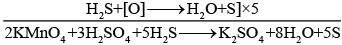5 moles of S2− ions react with 2 moles of KMnO4.
So, 1 mole of S2− ion will react with 2/5 moles of KMnO4

Q.12. Which of the following is amphoteric oxide?
Mn2O7, CrO3, Cr2O3, CrO, V2O5, V2O4
(i) V2O5, Cr2O3
(ii) Mn2O7, CrO3
(iii) CrO, V2O5
(iv) V2O5, V2O4

Ans. (a)
Solution.

V2O5 and Cr2O3 are amphoteric oxide because both react with alkalies as well as acids.

Q.13. Gadolinium belongs to 4f series. It’s atomic number is 64. Which of the following is the correct electronic configuration of gadolinium?
(i) [Xe] 4f75d16s2
(ii) [Xe] 4f65d26s2
(iii) [Xe] 4f86d2
(iv) [Xe] 4f95s1
Ans. (i)
Solution.

Gadolinium belongs to 4f series and has atomic number 64. The correct electronic configuration of gadolinium is
64Gd = 54[Xe]4f7 5d1 6s2
It has extra stability due to half-filled 4f subshell.

Q.14. Interstitial compounds are formed when small atoms are trapped inside the crystal lattice of metals. Which of the following is not the characteristic property of interstitial compounds?
(i) They have high melting points in comparison to pure metals.
(ii) They are very hard.
(iii) They retain metallic conductivity.
(iv) They are chemically very reactive.
Ans. (iv)
Solution.

Some of the important characteristics of interstitial compounds are as follows:
(i) They are very hard and rigid.
(ii) They have high melting point which are higher than those of the pure metals.
(iii) They show conductivity like that of the pure metal.
(iv) They acquire chemical inertness.

Q.15. The magnetic moment is associated with its spin angular momentum and orbital angular momentum. Spin only magnetic moment value of Cr3+ ion is ___________.
(i) 2.87 B.M.
(ii) 3.87 B.M.
(iii) 3.47 B.M.
(iv) 3.57 B.M.

Ans. (iv)
Solution.

The magnetic moment of an element is determined by the number of unpaired electrons and is calculated by using the ‘spin-only’ formula.
The formula for magnetic moment,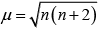Hence, magnetic moment value of Cr3 ion (3d3) is: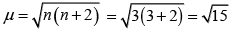= 3.87BM

Q.16. KMnO4 acts as an oxidising agent in alkaline medium. When alkaline KMnO4 is treated with KI, iodide ion  is oxidised to  _________.
(i) I2
(ii) IO
(iii)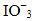(iv)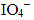Ans. (iii)
Solution.

KMnO4 acts as an oxidising agent in alkaline medium. When alkaline KMnO4 is treated with KI, iodide ion is oxidised to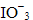Reaction 2KMnO4 + H2O+ KI → 2MnO4+ 2KOH + KIO3
Or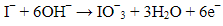Q.17. Which of the following statements is not correct?
(i) Copper liberates hydrogen from acids.
(ii) In its higher oxidation states,  manganese forms stable compounds with oxygen and fluorine.
(iii) Mn3+ and Co3+ are oxidising agents in aqueous solution.
(iv) Ti2+ and Cr2+ are reducing agents in aqueous solution.
Ans. (i)
Solution.
Copper lies below hydrogen in the electrochemical series and hence does not liberate H2 from acids, Therefore, option (i) is not correct, Other three options (ii, iii, iv) are correct.

Q.18. When acidified K2Cr2O7 solution is added to Sn2+ salts then Sn2+ changes to
(i) Sn
(ii) Sn3+
(iii) Sn4+
(iv) Sn+
Ans. (iii)
Solution.

When acidified K2CrsO solution is added to Sn2+ salts then Sn2+ changes to Sn4+. The reaction is given below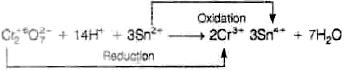Q.19. Highest oxidation state of manganese in fluoride is +4 (MnF4) but highest oxidation state in oxides is +7 (Mn2O7) because ____________.
(i) Fluorine is more electronegative than oxygen.
(ii) Fluorine does not possess d-orbitals.
(iii) Fluorine stabilises lower oxidation state.
(iv) In covalent compounds fluorine can form single bond only while oxygen forms double bond.
Ans. (iv)
Solution.

Highest oxidation state of manganese in fluoride is +4(MnF4) but highest oxidation state in Oxides is + 7(Mn2O7). The reason is that in covalent compounds fluorine can form single bond while oxygen forms double bond.

Q.20. Although Zirconium belongs to 4d transition series and Hafnium to 5d transition series even then they show similar physical and chemical properties because __________.
(i) Both belong to d-block.
(ii) Both have same number of electrons.
(iii) Both have similar atomic radius.
(iv) Both belong to the same group of the periodic table.
Ans. (iii)
Solution.

Due to lanthanoide contraction, Zr and Hf possess nearly same atomic and ionic radii i.e., Zr = 160 pm and Hf = 159 pm, Zr4+ = 79 pm and Hf4+ = 78 pm. Therefore, these two elements show similar properties (physical and chemical properties).

Q.21. Why is HCl not used to make the medium acidic in oxidation reactions of KMnO4 in acidic medium?
(i) Both HCl and KMnO4 act as oxidising agents.
(ii) KMnO4 oxidises HCl into Cl2 which is also an oxidising agent.
(iii) KMnO4 is a weaker oxidising agent than HCl.
(iv) KMnO4 acts as a reducing agent in the presence of HCl.
Ans. (ii)
Solution.

HCl is not used to make the medium acidic in oxidation reactions of KMnO4 in acidic medium. The reason is that if HCl is used, the oxygen produced from KMnO4 + HCl is partly utilised in oxidising HCl to CI2, which itself acts as an oxidising agent and partly oxidises the reducing agent.

MULTIPLE CHOICE QUESTIONS (TYPE - II)

Q.22. Generally transition elements and their salts are coloured due to the presence of unpaired electrons in metal ions. Which of the following compounds are coloured?
(i) KMnO4
(ii) Ce (SO4)2
(iii) TiCl4
(iv) Cu2Cl2
Ans. (i, ii)
Solution.

KMnO4 is coloured due to the charge transfer and not because of the presence of unpaired electrons. Similarly, oxidation state of Ce in Ce (SO4)2 is +4 with 4f0 electronic configuration. It is also coloured (yellow) due to charge transfer and not due to f−f transition.

Q.23. Transition elements show magnetic moment due to spin and orbital motion of electrons. Which of the following metallic ions have almost same spin only magnetic moment?
(i) Co2+
(ii) Cr2+
(iii) Mn2+
(iv) Cr3+
Ans. (i, iv)
Solution.

Electronic configuration of Co2+ = [Ar] 3d7; Number of unpaired electrons = 3
Electronic configuration of Cr2+ = [Ar] 3d4; Number of unpaired electrons = 4
Electronic configuration of Mn2+ = [Ar] 3d5; Number of unpaired electrons = 5
Electronic configuration of Cr3+ = [Ar] 3d3; Number of unpaired electrons = 3
Hence, it is clearly seen that both Co2+ and Cr3+ have same number of unpaired electrons.
i.e., 3, so they have almost same spin only magnetic moment.

Q.24. In the form of dichromate, Cr (VI) is a strong oxidising agent in acidic medium but Mo (VI) in MoO3 and W (VI) in WO3 are not because ___________.
(i) Cr (VI) is more stable than Mo(VI) and W(VI).
(ii) Mo(VI) and W(VI) are more stable than Cr(VI).
(iii) Higher oxidation states of heavier members of group-6 of transition series are more stable.
(iv) Lower oxidation states of heavier members of group-6 of transition series are more stable.
Ans. (ii, iii)
Solution.

In d-block elements, for heavier elements, the higher oxidation states are more stable. Hence, Mo (VI) and W(VI) are more stable than Cr (VI). Due to this, Cr (VI) in the form of dichromate is a stronger oxidising agent in acidic medium whereas MoO3 and WO3 are not.

Q.25. Which of the following actinoids show oxidation states upto +7?
(i) Am
(ii) Pu
(iii) U
(iv) Np
Ans. (ii, iv)
Solution.

The oxidation states of the given actinoids are as follows:
(i) Americium (Z = 95); Electronic configuration = [Rn] 5f76d07s2
Oxidation states shown by Am = + 3, + 4, + 5, + 6.
(ii) Plutonium (Z = 94); Electronic configuration = [Rn] 5f66d07s2
Oxidation states shown by Pu = + 3, + 4, + 5, + 6, + 7.
(iii) Uranium (Z = 92); Electronic configuration = [Rn] 5f36d17s2
Oxidation states shown by U = + 3, + 4, + 5, + 6.
(iv) Neptunium (Z = 93); Electronic configuration = [Rn] 5f46d17s2
Oxidation states shown by Np = + 3, + 4, + 5. + 6, + 7.

Q.26. General electronic configuration of actionoids is (n–2)f1–14 (n-1)d0–2ns2. Which of the following actinoids have one electron in 6d orbital?
(i) U (Atomic number. 92)
(ii) Np (Atomic number.93)
(iii) Pu (Atomic number. 94)
(iv) Am (Atomic number. 95)
Ans. (i, ii)
Solution.

General electronic configuration of actinoids is (n−1)f1−14(n−1)d0−2ns2. U and Np each have one electron in 6d orbital.
(i) Uranium (Z = 92); Electronic configuration = [Rn] 5f36d17s2
(ii) Neptunium (Z = 93); Electronic configuration = [Rn] 5f46d17s2
(iii) Plutonium (Z = 94); Electronic configuration = [Rn] 5f66d07s2
(iv) Americium (Z = 95); Electronic configuration = [Rn] 5f76d07s2

Q.27. Which of the following lanthanoids show +2 oxidation state besides the characteristic oxidation state +3 of lanthanoids?
(i) Ce
(ii) Eu
(iii) Yb
(iv) Ho
Ans. (ii, iii)
Solution.

(i) Cerium (Z = 57)  ⇒ Electronic configuration = [Xe]4f55d°6s2
Oxidation state of Ce = +3, + 4
(ii) Europium (Z = 63) ⇒ Electronic configuration = [Xe]4f75d°6s2
Oxidation state of Eu = +2, + 3
(iii) Ytterbium (Z = 70) ⇒ Electronic configuration = [Xe]4f145d°6s2
Oxidation state of Yb = + 2, + 3
(iv) Holmium (Z = 67) Þ Electronic configuration = [Xe]4f115d°6s2
Oxidation state of Ho = +3

Q.28. Which of the following  ions show higher spin only magnetic moment value?
(i) Ti3+
(ii) Mn2+
(iii) Fe2+
(iv) Co3+
Ans. (ii, iii)
Solution.

As, Ti3+ = [Ar] 3d1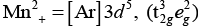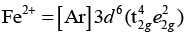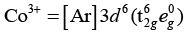Crystal field splitting energy (CFSE) is high in Co3+ and electrons pair up in t2g. Hence, only Fe2+ and Mn2+ show higher spin magnetic moment value.

Q.29. Transition elements form binary compounds with halogens. Which of the following elements will form MFtype compounds?
(i) Cr
(ii) Co
(iii) Cu
(iv) Ni
Ans. (i, ii)
Solution.

Transition elements such as Cr and Co form binary compounds with halogens, i.e., CrF3 and CoF3 whereas Cu and Ni do not form CuF3 and NiF3.

Q.30. Which of the following will not act as oxidising agents?
(i) CrO3
(ii) MoO3
(iii) WO3
(iv)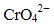Ans. (ii, iii)
Solution.

A species can act as oxidising agent only when metal is present in high oxidation state but its lower oxidation state shows stability. As higher oxidations states of W and Mo are more stable, therefore they will not act as oxidising agents.

Q.31. Although +3 is the characteristic oxidation state for lanthanoids but cerium also shows +4 oxidation state because ________.
(i) It has variable ionisation enthalpy
(ii) It has a tendency to attain noble gas configuration
(iii) It has a tendency to attain f0 configuration
(iv) It resembles Pb4+
Ans. (ii, iii)
Solution.

Electronic configuration of 58Ce = 54[Xe] 4f25d06s2.
Therefore, electronic configuration of Ce4+= 54[Xe] 4f0.
Thus, it has a tendency to attain noble gas configuration and attain fconfiguration.

Q.32. Why does copper not replace hydrogen from acids?
Ans. Copper does not replace hydrogen from acids because Cu has positive Eo (= +0.34 V) value, i.e., less reactive than hydrogen which has electrode potential of l0.00V.

Q.33. Why Eº values for Mn, Ni and Zn are more negative than expected?

Ans. Negative values of Mn2+ and Zn2+ are related to stabilities of half-filled and completely filled configurations respectively. But for Ni2+, Eo value is related to the highest negative enthalpy of hydration.
Hence, Eo values for Mn, Ni and Zn are more negative than expected.

Q.34. Why first ionisation enthalpy of Cr is lower than that of  Zn?
Ans. Ionisation enthalpy of Cr is less than that of Zn because Cr has stable d5 configuration. In case of zinc, electron comes out from completely filled 4s-orbital. So, removal of electron from zinc requires more energy as compared to the chromium.

Q.35. Transition elements show high melting points. Why?

Ans. Transition elements show high melting points, due to the involvement of greater number of electrons in the interatomic bonding from (n-1) d-orbitals in addition to ns electrons in forming metallic bond.

Q.36. When Cu2+ ion is treated with KI, a white precipitate is formed. Explain the reaction with the help of chemical equation.

Ans. When Cu2+ ion is treated with KI, it produces Cu2I2 white precipitate in the final product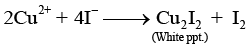Q.37. Out of Cu2Cl2 and CuCl2, which is more stable and why?

Ans. Among Cu2CI2 and CuCI2, CuCI2 is more stable. Stability of complex can be explained on the basis of stability of oxidation state of copper. Stability of Cu2+ (aq) ion is due to hgher negative value of Δhyd Hof Cu2+ (aq) than Cu+ which is enough to compensates for the second ionisation enthalpy of Cu.

Q.38. When a brown compound of manganese (A) is treated with HCl it gives a gas (B). The gas taken in excess, reacts with NH3 to give an explosive compound (C). Identify compounds A, B and C.

Ans. MnO2 is the brown compound of Mn which reacts with HCl to give Cl2 gas. This gas forms an explosive compound NCl3 when treated with NH3. Thus, A = MnO2; B = CI2; C = NCl3 and reactions are as follows:
(i) MnO2 +HCl → MnCl+ Cl2 + 2H2O
(ii)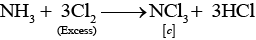Q.39. Although fluorine is more electronegative than oxygen, but the ability of oxygen to stabilise higher oxidation states exceeds that of fluorine. Why?

Ans. Oxygen can form multiple bonds with metals, while fluorine can't form multiple bonds. Hence, oxygen has more ability to stabilize higher oxidation state rather than fluorine.

Q.40. Although Cr3+ and Co2+ ions have same number of unpaired electrons but the magnetic moment of Cr3+ is 3.87 B.M. and that of Co2+ is 4.87 B.M. Why?

Ans. Magnetic moment of any metal ion can be decided on the basis of spin as well as orbital contribution of electrons. Due to symmetrical electronic configuration, there is no orbital contribution in Cr3+ ion. However, appreciable orbital contribution takes place in Co2+ ion.

Q.41. Ionisation enthalpies of Ce, Pr and Nd are higher than Th, Pa and U. Why?

Ans. Ce, Pr and Nd are lanthanoids and have incomplete 4f shell while Th, Pa and U are actinoids and have incomplete 5f shell.
In the beginning, when 5f-orbitals begin to be occupied, they will penetrate less into the inner core of electrons. The 5f-electrons will therefore, be more effectively shielded from the nuclear charge than 4f electrons of the corresponding lanthanoids.
Therefore, outer electrons are less firmly held and they are available for bonding in the actinoids.

Q.42. Although Zr belongs to 4d and Hf belongs to 5d transition series but it is quite difficult to separate them. Why?
Ans. Separation of Zr and Hf is quite difficult because of lanthanoid contraction. Due to lanthanoid contraction, they have almost same size (Zr = 160 pm and Hf = 159 pm) and thus, similar chemical properties. That's why it is very difficult to separate them by chemical methods.

Q.43. Although +3 oxidation states is the characteristic oxidation state of lanthanoids but cerium shows +4 oxidation state also. Why?

Ans. It is due to the fact that after losing one more electron Ce acquires stable 4f0 electronic configuration. So, Ce shows +4 oxidation state also alongwith +3 oxidation state.

Q.44. Explain why does colour of KMnO4 disappear when oxalic acid is added to its solution in acidic medium.
Ans. When oxalic acid is added to acidic solution of KMnO4, its colour disappear due to reduction of MnO4 ion to Mn2+. Chemical reaction occurring during this neutralisation reaction is as follows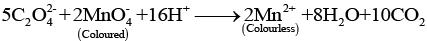Q.45. When orange solution containing  ion is treated with an alkali, a yellow solution is formed and when H+ ions are added to yellow solution, an orange solution is obtained. Explain why does this happen?

Ans. When orange solution containing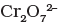ion is treated with an alkali, a yellow solution ofis obtained. In the same way, when H+ ions are added to yellow solution, an orange solution is obtained due to interconversion.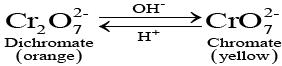Q.46. A solution of KMnO4 on reduction yields either a colourless solution or a brown precipitate or a green solution depending on pH of the solution. What different stages of the reduction do these represent and how are they carried out?

Ans. Oxidising behaviour of KMnO4 depends on pH of the solution.
In acidic medium (pH < 7)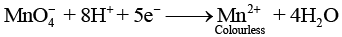In alkaline medium (pH > 7)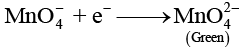In neutral medium (pH = 7)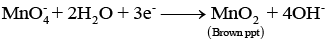Q.47. The second and third rows of transition elements resemble each other much more than they resemble the first row. Explain why?
Ans. Due to lanthanoid contraction, the atomic radii of the second and third row transition elements is almost same. So, they resemble each other much more as compared to first row elements and show similar character.

Q.48. Eº of Cu is + 0.34V while that of Zn is – 0.76V. Explain.
Ans. Eº value of Cu is positive because of the fact that sum of sublimation enthalpy and ionisation enthalpy to convert Cu(s) to Cu2+ (aq) is so high that it is not compensate by its hydration enthalpy. Eº value for Zn is negative because of the fact that after removal of electrons from 4s orbital, stable 3d10 configuration is obtained.

Q.49. The halides of transition elements become more covalent with increasing oxidation state of the metal. Why?

Ans. As the oxidation state increases, size of the ion of transition element decreases. As per Fajan's rule, as the size of metal ion decreases, covalent character of the bond formed increases.
Therefore, the halides of transition elements become more covalent with increasing oxidation state of the metal.

Q.50. While filling up of electrons in the atomic orbitals, the 4s orbital is  filled before the 3d orbital but reverse happens during the ionisation of the atom. Explain why?
Ans. Filling up of electrons follows n + l rule. Here 4s has lower energy than 3d orbital. After the orbitals are filled 4s goes beyond 3d, i.e., 4s is farther from nucleus than 3d. So, electron from 4s is removed earlier than from 3d.

Q.51. Reactivity of transition elements decreases almost regularly from Sc to Cu. Explain.

Ans. Reactivity of transition elements depends mostly upon their ionisation enthalpies. As we move from left to right in the periodic table (Se to Cu), ionisation enthalpies increase almost regularly. Hence, their reactivity decreases almost regularly from Se to Cu.

MATCHING TYPE

Note : Match the items of Column I and Column II in the following questions.
Q.52. Match the catalysts given in Column I with the processes given in Column II.

 Column I (Catalyst) Column II (Process) (i) Ni in the presence of hydrogen (a) Zieglar Natta catalyst (ii) Cu2Cl2 (b) Contact process (iii) V2O5 (c) Vegetable oil to ghee (iv) Finely divided iron (d) Sandmeyer reaction (v) TiCl4 + Al (CH3)3 (e) Haber’s Process (f) Decomposition of KClO3

Ans. (i) → (c), (ii) → (d), (iii) → (b), (iv) → (e), (v) → (a)
Solution.

 Column I (Catalyst) Column II (Process) (i) Ni in the presence of hydrogen (c) Vegetable oil to ghee (ii) Cu2Cl2 (d) Sandmeyer reaction (iii) V2O5 (b) Contact process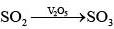(iv) Finely divided iron (e) Haber’s Process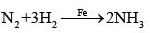(v) TiCl4 + Al (CH3)3 (a) Zieglar Natta catalyst

Q.53. Match the compounds/elements given in Column I with uses given in Column II.

 Column I (Compound/element) Column II (Use) (i) Lanthanoid oxide (a) Production of iron alloy (ii) Lanthanoid (b) Television screen (iii) Misch metal (c) Petroleum cracking (iv) Magnesium based alloy is constituent of (d) Lanthanoid metal + iron (v) Mixed oxides of lanthanoids are employed (e) Bullets (f) In X-ray screen

Ans. (i) → (b), (ii) → (a), (iii) → (d), (iv) → (e), (v) → (c)
Solution.

 Column I (Compound/element) Column II (Use) (i) Lanthanoid oxide (b) Television screen (ii) Lanthanoid (a) Production of iron alloy (iii) Misch metal (d) Lanthanoid metal + iron (iv) Magnesium based alloy is constituent of (e) Bullets (v) Mixed oxides of lanthanoids are employed (c) Petroleum cracking

Q.54. Match the properties given in Column I with the metals given in Column II.

 Column I (Property) Column II (Metal) (i) An element which can show +8 oxidation state (a) Mn (ii) 3d block element  that can show upto +7 oxidation state (b) Cr (iii) 3d block element with highest melting point (c) Os (d) Fe

Ans. (i) → (c), (ii) → (a), (iii) → (b)
Solution.
(i) Osmium is an element which can show +8 oxidation state.
(ii) 3d block element that can show upto + 7 oxidation state is manganese.
(iii) 3d block element with highest melting point is chromium.

Q.55. Match the statements given in Column I with the oxidation states given in Column II.

 Column I Column II (i) Oxidation state of Mn in MnO2 is (a) +2 (ii) Most stable oxidation state of Mn is (b) +3 (iii) Most stable oxidation state of Mn in oxides is (c) +4 (iv) Characteristic oxidation state of lanthanoids is (d) + 5 (e) + 7

Ans. (i)→ (c), (ii) → (a), (iii) → (e), (iv) → (b)
(i) Oxidation state of Mn in MnO2 is + 4.
(ii) Most stable oxidation state of Mn is +2
(iii) Most stable oxidation state of Mn in oxides is +7.
(iv) Characteristic oxidation state of lanthanoids is + 3.

Q.56. Match the solutions given in Column I and the colours given in Column II.

 Column I Column II (Aqueous solution of salt) (Colour) (i) FeSO4.7H2O (a) Green (ii) NiCl2.4H2O (b) Light pink (iii) MnCl2.4H2O (c) Blue (iv) CoCl2.6H2O (d) Pale green (v) Cu2Cl2 (e) Pink (f) Colourless

Ans. (i) → (d), (ii) → (a), (iii) → (b), (iv) → (e), (v) → (f)

 Column I Column II (Aqueous solution of salt) (Colour) (i) FeSO4.7H2O (d) Pale green (ii) NiCl2.4H2O (a) Green (iii) MnCl2.4H2O (b) Light pink (iv) CoCl2.6H2O (e) Pink (v) Cu2Cl2 (f) Colourless

Q.57. Match the property given in Column I with the element given in Column II.

 Column I (Property) Column II (Element) (i) Lanthanoid which shows +4 oxidation state (a) Pm (ii) Lanthanoid which can show +2 oxidation state (b)  Ce (iii) Radioactive lanthanoid (c)  Lu (iv) Lanthanoid which has 4f7 electronic configuration in +3 oxidation state (d)  Eu (v) Lanthanoid which has 4f14 electronic configuration in +3 oxidation state (e) Gd (f)  Dy

Ans. (i) → (b), (ii) → (d), (iii) → (a), (iv) → (e), (v) → (c)
Solution.
(i) Lanthanoid which shows +4 oxidation state is cerium.
58Ce = [Xe] 4f2 5dº 6s2;Oxidation state = +3, +4
(ii) Lanthanoid which can show +2 oxidation states is europium.
63Eu =[Xe] 4f7 5dº 6s2;Oxidation state = + 2, +3
(iv) Lanthnoid which has 4f7 electronic configuration in +3 oxidation state is gadolinium.
64Gd =[Xe] 4f7 5d1 6s2; Oxidation state = +3
(v)  Lanthanoid which has 4f14 electronic configuration in +3 oxidation state is lutetium
71Lu = [Xe] 4f14 5d1 6s2;Oxidation state = +3

Q.58. Match the properties given in Column I with the metals given in Column II.

 Column I (Property) Column II (Metal) (i) Element with highest second ionisation enthalpy (a) Co (ii) Element with highest third ionisation enthalpy (b) Cr (iii) M in M (CO)6 is (c) Cu (iv) Element with highest heat of  atomisation (d) Zn (e) Ni

Ans. (i) → (c), (ii) → (d), (iii) → (b), (iv) → (a)
Solution.
(i) Cu+ = 3d10 which is very stable configuration due to fully filled orbitals. Hence, removal of second electron requires very high energy.
(ii) Zn2+ = 3d10 which is very stable configuration. Hence, removal of third electron requires very high energy,
(iii) Metal carbonyl with formula M(CO)6 is Cr(CO)6.
(iv) Nickel is the element with highest heat of atomisation.

ASSERTION AND REASON TYPE

Note : In the following questions a statement of Assertion followed by a statement of Reason is given. Choose the correct answer out of the following choices.
(i) Both assertion and reason are true, and reason is the correct explanation of the assertion.
(ii) Both assertion and reason are true but reason is not the correct explanation of assertion.
(iii) Assertion is not true but reason is true.
(iv) Both assertion and reason are false.
Q.59. Assertion : Cu2+ iodide is not known.
Reason : Cu2+ oxidises I – to iodine.
(i) Both assertion and reason are true, and reason is the correct explanation of the assertion.
(ii) Both assertion and reason are true but reason is not the correct explanation of assertion.
(iii) Assertion is not true but reason is true.
(iv) Both assertion and reason are false.
Ans. (i)
Solution.
Copper (II) iodide (CuI2) is not known because Cu2+ oxidises I to Iodine.

Q.60. Assertion : Separation of Zr and Hf  is difficult.
Reason : Because Zr and Hf  lie in the same group of the periodic table.
(i) Both assertion and reason are true, and reason is the correct explanation of the assertion.
(ii) Both assertion and reason are true but reason is not the correct explanation of assertion.
(iii) Assertion is not true but reason is true.
(iv) Both assertion and reason are false.
Ans. (ii)
Solution.
Separation of Zr and Hf is difficult; it is not because of they lie in the same group of Periodic Table but due to lanthanoid contraction which causes almost similar radii of both of them.

Q.61. Assertion : Actinoids form relatively less stable complexes as compared to lanthanoids.
Reason : Actinoids can utilise their 5f orbitals along with 6d orbitals in bonding but lanthanoids do not use their 4f orbital for bonding.
(i) Both assertion and reason are true, and reason is the correct explanation of the assertion.
(ii) Both assertion and reason are true but reason is not the correct explanation of assertion.
(iii) Assertion is not true but reason is true.
(iv) Both assertion and reason are false.
Ans. (iii)
Solution.
Actinoids form relatively more stable complexes as compared to lanthanoids because of actinoids can utilise their 5f orbitals along with 6d orbitals in bonding but lanthanoids do not use their 4f orbitals for bonding.

Q.62. Assertion : Cu cannot liberate hydrogen from acids.
Reason : Because it has positive electrode potential.
(i) Both assertion and reason are true, and reason is the correct explanation of the assertion.
(ii) Both assertion and reason are true but reason is not the correct explanation of assertion.
(iii) Assertion is not true but reason is true.
(iv) Both assertion and reason are false.
Ans. (i)
Solution.
Cu can not liberate hydrogen from acids because it has positive electrode potential. Metals having negative value of electrode potential liberate H2 gas.

Q.63. Assertion : The highest oxidation state of osmium is +8.
Reason : Osmium is a 5d-block element.
(i) Both assertion and reason are true, and reason is the correct explanation of the assertion.
(ii) Both assertion and reason are true but reason is not the correct explanation of assertion.
(iii) Assertion is not true but reason is true.
(iv) Both assertion and reason are false.
Ans. (ii)
Solution.
The highest oxidation state of osmium is +8 due to its ability to expand their octet by using its all 8 electrons (2 from 6s and 6 from 5d).

Q.64. Identify A to E and also explain the reactions involved.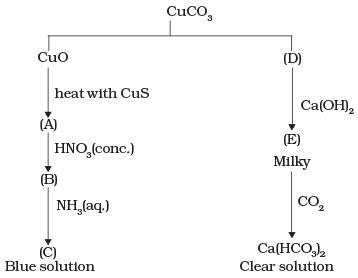Ans. The substances from A to E are
A = Cu; B = Cu (NO3)2; C = [Cu(NH3)4]2+; D = CO2; E = CaCO3
Reactions:
(i)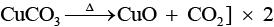(ii)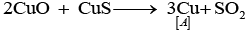(iii)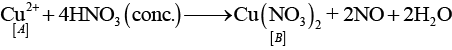(iv)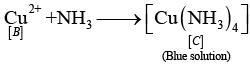(v)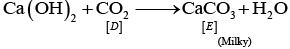(vi) CaCO3 + H2O + CO→ Ca HCO3)2

Q.65. When a chromite ore (A) is fused with sodium carbonate in free excess of air and the product is dissolved in water, a yellow solution of compound (B) is obtained. After treatment of this yellow solution with sulphuric acid, compound (C) can be crystallised from the solution. When compound (C) is treated with KCl, orange crystals of compound (D) crystallise out. Identify A to D and also explain the reactions.
Ans. K2Cr2O7 is an orange compound. It is formed when Na2Cr2O7 reacts with KCI. In acidic medium, yellow coloured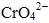(chromate ion) changes into dichromate.
The given process is the preparation method of potassium dichromate from chromite ore.
A = FeCr2O4; B = Na2CrO4; C = Na2Cr2O7; D = K2Cr2O7.
(i)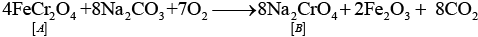(ii) 2Na2CrO4 + 2H+ → Na2Cr2O7 + 2Na+ + H2O
(iii)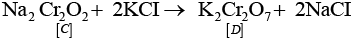Q.66. When an oxide of manganese (A) is fused with KOH in the presence of an oxidising agent and dissolved in water, it gives a dark green solution of compound (B). Compound (B) disproportionates in neutral or acidic solution to give purple compound (C). An alkaline solution of compound (C) oxidises potassium iodide solution to a compound (D) and compound (A) is also formed. Identify compounds A to D and also explain the reactions involved.
Ans. It is the method of preparation of potassium permanganate (purple).
Thus, (A) =MnO2
(B) = K2MnO4
(C) =KMnO4
(D) = KIO3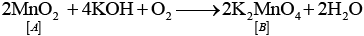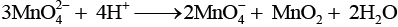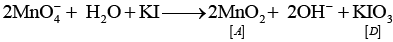Q.67. On the basis of Lanthanoid contraction, explain the following :
(i) Nature of bonding in La2O3 and Lu2O3.
(ii) Trends in the stability of oxo salts of lanthanoids from La to Lu.
(iii) Stability  of the complexes of lanthanoids.
(iv) Radii of 4d and 5d block elements.
(v) Trends in acidic character of lanthanoid oxides.
Ans. (i) As the size decreases covalent character increases. Therefore, La2O3 is more ionic and Lu2O3 is more covalent.
(ii) As the size decreases from La to Lu. stability of oxo salts also decreases.
(iii) Stability of complexes increases as the size of lanthanoids decreases.
(iv) Radii of 4d and 5d-block elements will be almost same.
(v) Acidic character of oxides increases from La to Lu.

Q.68. (a) Answer the following questions :
(i) Which element of the first transition series has highest second ionisation enthalpy?

(ii) Which element of the first transition series has  highest third ionisation enthalpy?
(iii) Which element of the first transition series has  lowest enthalpy of atomisation?
(i) Carbonyl M (CO)5
(ii) MO3F

Ans. (a) (i) Cu because the electronic configuration of Cu is 3d104s1. So, second electron needs to be removed from completely filled d-orbital which is very difficult.
(ii) Zinc because of electronic configuration of Zn = 3d104s2 and Zn2+ = 3d10 which is fully filled and hence is very stable. Removal of third electron requires very high energy.
(iii) Zinc, because of it has completely filled 3d subshell and no unpaired electron is available for metallic bonding.
(b) (i) Carbonyl M(CO)5 is Fe(CO)5
According to EAN rule, the effective number of a metal in a metal carbonyl is equal to the atomic number of nearest inert gas EAN is calculated as
EAN = number of electrons in metal +2 x (CO)
= atomic number of nearest inert gas
In M(CO)5 = x + 2 x (5) = 36 (Kr is the nearest inert gas)
x = 26 (atomic number of metal)
So, the metal is Fe (iron)
(ii) MO3F is MnO3F.
In MO3F
Let us assume that oxidation state of M is x
x + 3 x (−2) + (−1) = O
or, x = +7 i.e., M is in + 7 oxidation state of +7.
Hence, the given compound is MnO3F.

Q.69. Mention the type of compounds formed when small atoms like H, C and N get trapped inside the crystal lattice of transition metals. Also give physical and chemical characteristics of these compounds.
Ans. When small atoms like H, C and N get trapped inside the crystal lattice of transition metals.
(a) Such compounds are called interstitial compounds.
(b) Their characteristic properties are
(i) They have high melting points, higher than those of pure metals.
(ii) They are very hard
(ii) They retain metallic conductivity.
(iv) They are chemically inert.

Q.70. (a) Transition metals can act as catalysts because these can change their oxidation state. How does Fe(III) catalyse the reaction between iodide and persulphate ions?
(b) Mention any three processes where transition metals act as catalysts.
Ans. (a) Reaction between iodide and persulphate ions is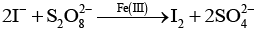Role of Fe (III) ions -------------- 2Fe3+ + 2I- → 2Fe2+ + I2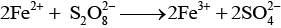(b) (i) Vanadium (V) oxide used in contact process for oxidation of SO2 to SO3.
(ii) Finely divided iron in Haber's process in conversion of N2 and H2 to NH3.
(iii) MnO2 in preparation of oxygen from KClO3.

Q.71. A violet compound of manganese (A) decomposes on heating to liberate oxygen and compounds (B) and (C) of manganese are formed. Compound (C) reacts with KOH in the presence of potassium nitrate to give compound (B). On heating compound (C) with conc. H2SO4 and NaCl, chlorine gas is liberated and a compound (D) of manganese along with other products is formed.
Identify compounds A to D and also explain the reactions involved.
Ans. Since, compound (C) on treating with conc. H2SO4 and NaCl gives Cl2 gas, so it is manganese dioxide (MnO2). It is obtained along with MnO42− when KMnO4(violet) is heated
Thus, (A) =KMnO4
(B) = K2MnO4
(C) = MnO2
(D) =MnCl2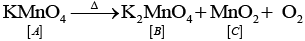2MnO2 + 4KOH2 + O → 2K2 MnO4 + 2H2O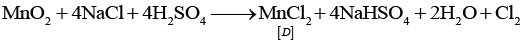Offer running on EduRev: Apply code STAYHOME200 to get INR 200 off on our premium plan EduRev Infinity!

,

,

,

,

,

,

,

,

,

,

,

,

,

,

,

,

,

,

,

,

,

;